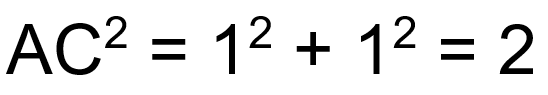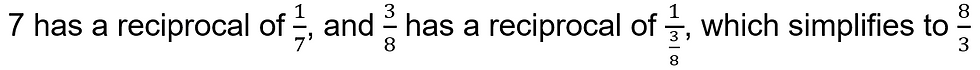top of page
Search
• Wim G

# Numbers and Language

Updated: May 20, 2022

## Vocabulary for different types of numbers

There are numerous ways to classify a square. It's a quadrilateral, a polygon, and a two-dimensional shape all rolled into one. Numbers, like shapes, can be categorised in a variety of ways. A collection of some of the most common numbers is listed below.

## Natural numbers

'One, two, three, four,...' a child learns to count. These are also known as whole numbers or counting numbers. "I am three," the child will say, or "I live at number 73."

Natural numbers are a collection of numbers that includes the number 0.

ℕ = 0, 1, 2, 3, 4,... is a set of natural numbers.

## Integers

On a chilly day, the temperature at 10 p.m. may be 4 °C. If the temperature drops another 6 degrees Celsius, it is 'below zero,' or -2 degrees Celsius.

If you have a \$200 bank overdraft, this can be shown as -\$200.

ℤ =..., -3, --2, 1, 0, 1, 2, 3,... is a set of integers. As a result, is an extension of ℕ.

Every integer is a natural number.

## Rational numbersThe set of rational numbers is an extension of the set of integers.

## Irrational numbers

Irrational numbers are not rational numbers since they cannot be stated as fractions.The length of the hypotenuse AC is obtained using Pythagoras' formula in the diagram to the right:AC = √2 AC = 1.41421356

The digits in this number do not duplicate themselves in any way. All irrational numbers have this property.

Another irrational number you will encounter is π(pi). It is the ratio of a circle's circumference to its diameter length. The digits continue continuously, never repeating themselves in any specific pattern, even when rounded to 3.142.

## Real numbers

The set of real numbers consists of the set of rational numbers plus irrational numbers.

## Prime numbers

A prime number has only two factors: one and the number itself. (It's worth noting that 1 is not a prime number.)

## Reciprocal

When you divide the number 1 by a number, the reciprocal of that number is produced.## Square numbersThis can be written as '1 squared' or '1 to the power of 2'.When you multiply an integer (whole number) by itself, you get a square number.

1, 4, 9, and 16 are all square numbers in the instances above.

## Cube numbersThis can be translated as "one cubed" or "one raised to the power of three."When you multiply an integer by itself and then by itself again, you get a cube number.

1, 8, 27, and 64 are all cube numbers in the instances above.

## Factors

All the numbers that divide perfectly into 12 are factors of 12: These are 1, 2, 3, 4, 6, and 12.

## Prime factors

1, 2, 3, 4, 6, and 12 are the factors of 12.

Since 2 and 3 are prime numbers, they are prime factors of 12.

Examples:

a) First find the prime factors of 18 and then express it as a product of prime numbers:b) First find the prime factors of 24 and then express it as a product of prime numbers:c) First find the prime factors of 75 and then express it as a product of prime numbers:## Highest common factor

The prime factors of 12 are 2 × 2 × 3.

The prime factors of 18 are 2 × 3 × 3.

This means the highest common factor (HCF) that can be seen by inspection will be

2 × 3, i.e., 6.

## Multiples

5, 10, 15, 20, and so on are all multiples of five.

Because 6 is the smallest number divisible by 2 and 3, it is the lowest common multiple (LCM) of 2 and 3.

3 and 5 have an LCM of 15.

6 and 10 have an LCM of 30.

## Square roots

There are 16 squares in the square presented. It has four-unit-long sides. The square root of 16 is therefore 4.This can be expressed as √16 = 4. Because 4 × 4 = 16, 4 is the square root of 16.

However, because -4× -4 is also = 16, -4 is also the square root of 16.

By convention, 16 denotes the "positive square root of 16," therefore 16 = 4, but the square root of 16 is 4 (i.e., +4 or -4).

Because any number squared is positive, -16 has no square root.

## Cube roots

The cube pictured has two-unit sides and takes up eight cubic units of space. (In other words, 2 × 2 × 2.)The cube root of 8 is therefore 2. It can also be written as ∛8 = 2.Because 4 × 4 × 4 = 64, ∛64 = 4.

Note that ∛64 ≠ -4 , as -4 × -4 × -4 = -64, but ∛(-64) = -4.